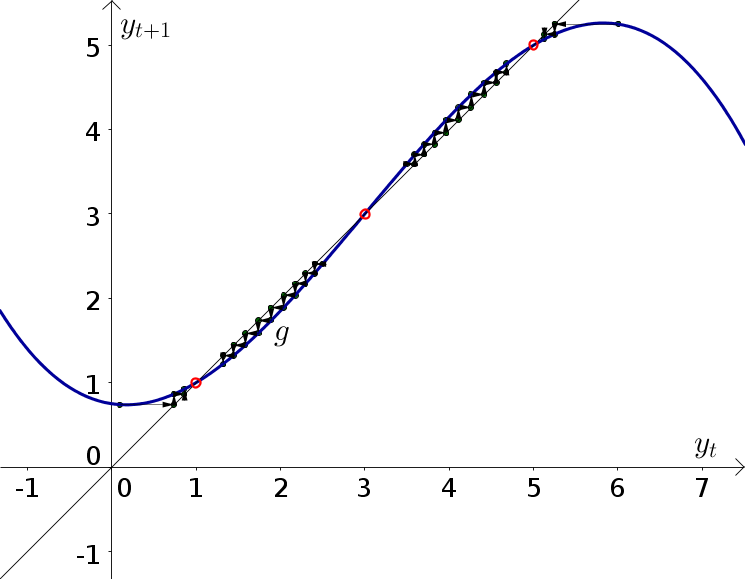# Math Insight

### Image: Discrete dynamical system example function 2, with cobwebbingThe function $y_{n+1}=g(y_n)=y_n - 0.05 (y_n - 1) (y_n - 3) (y_n - 5)$ is plotted in blue along with the diagonal line $y_{n+1}=y_n$. Equilibria of the dynamical system are illustrated by the red circles at the points where the two graphs intersect. Cobwebbing is indicated by the arrows.

Source image file: discrete_dynamical_system_example_function_2_cobweb.ggb
Source image type: Geogebra